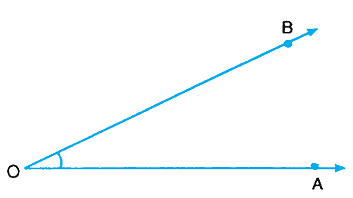# Angle

An angle is a figure consisting of two rays starting from a common point. An angle is measured in degrees.

The angle may be named as angle AOB or angle BOA or simply angle O, and is written as ∠ΑΟΒ or ∠ΒΟΑ or ∠Ο.Angle obtained by two opposite rays is called a straight angle

An angle of 90° is called a right angle. Two lines or rays making a right angle with each other are called perpendicular lines.

An angle less than 90° is called an acute angle. An angle greater than 90° but less than 180° is called an obtuse angle.